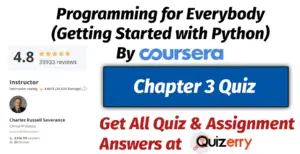# Chapter 3 (Quiz Answers) | Week-5 | Programming for Everybody (Getting Started with Python) By Coursera

###CourseraProgramming for Everybody (Getting Started with Python)Week 5Chapter 3Graded Quiz • 30 min

1. What do we do to a Python statement that is immediately after an if statement to indicate that the statement is to be executed only when the if statement is true?
• Underline all of the conditional code
• Indent the line below the if statement
• Start the statement with a “#” character
• Begin the statement with a curly brace {

2. Which of these operators is not a comparison / logical operator?
• ==
• <
• >=
• =
• !=

3. What is true about the following code segment:
`if  x == 5 :    print('Is 5')    print('Is Still 5')    print('Third 5')`
• Depending on the value of x, either all three of the print statements will execute or none of the statements will execute
• The string ‘Is 5’ will always print out regardless of the value for x.
• The string ‘Is 5’ will never print out regardless of the value for x.
• Only two of the three print statements will print out if the value of x is less than zero.

4. When you have multiple lines in an if block, how do you indicate the end of the if block?
• You omit the semicolon ; on the last line of the if block
• You put the colon : character on a line by itself to indicate we are done with the if block
• You use a curly brace { after the last line of the if block
• You de-indent the next line past the if block to the same level of indent as the original if statement

5. You look at the following text:
`if x == 6 :    print('Is 6')    print('Is Still 6')    print('Third 6')`
It looks perfect but Python is giving you an ‘Indentation Error’ on the second print statement. What is the most likely reason?
• Python thinks ‘Still’ is a mis-spelled word in the string
• In order to make humans feel inadequate, Python randomly emits ‘Indentation Errors’ on perfectly good code – after about an hour the error will just go away without any changes to your program
• Python has reached its limit on the largest Python program that can be run
• You have mixed tabs and spaces in the file

6. What is the Python reserved word that we use in two-way if tests to indicate the block of code that is to be executed if the logical test is false?
• break
• toggle
• switch
• else

7. What will the following code print out?
`x = 0if x < 2 :    print('Small')elif x < 10 :    print('Medium')else :    print('LARGE')print('All done')`
• LARGE

All done

• Small
• Small

Medium

LARGE
All done

• Small

All done

8. For the following code,
`if x < 2 :    print('Below 2')elif x >= 2 :     print('Two or more')else :    print('Something else')`
What value of ‘x’ will cause ‘Something else’ to print out?
• x = 2.0
• x = -2.0
• This code will never print ‘Something else’ regardless of the value for ‘x’
• x = 22

9. In the following code (numbers added) – which will be the last line to execute successfully?
`(1)   astr = 'Hello Bob'(2)   istr = int(astr)(3)   print('First', istr)(4)   astr = '123'(5)   istr = int(astr)(6)   print('Second', istr)`
• 1
• 3
• 2
• 6

10. For the following code:
`astr = 'Hello Bob'istr = 0try:    istr = int(astr)except:    istr = -1`
What will the value be for istr after this code executes?
• It will be a random number depending on the operating system the program runs on
• -1
• It will be the ‘Not a number’ value (i.e. NaN)
• false

The above questions are from “Programming for Everybody (Getting Started with Python)” You can discover all the refreshed questions and answers related to this on the “Programming for Everybody (Getting Started with Python) By Coursera” page. If you find the updated questions or answers, do comment on this page and let us know. We will update the answers as soon as possible.

Do Not Only Use These Quizzes For Getting Certificates.You Can Take Help From These Quizzes Answer. All Quizzes & Contents Are Free Of Charge. ✅

If You Want Any Quiz Answers Then Please Contact Us